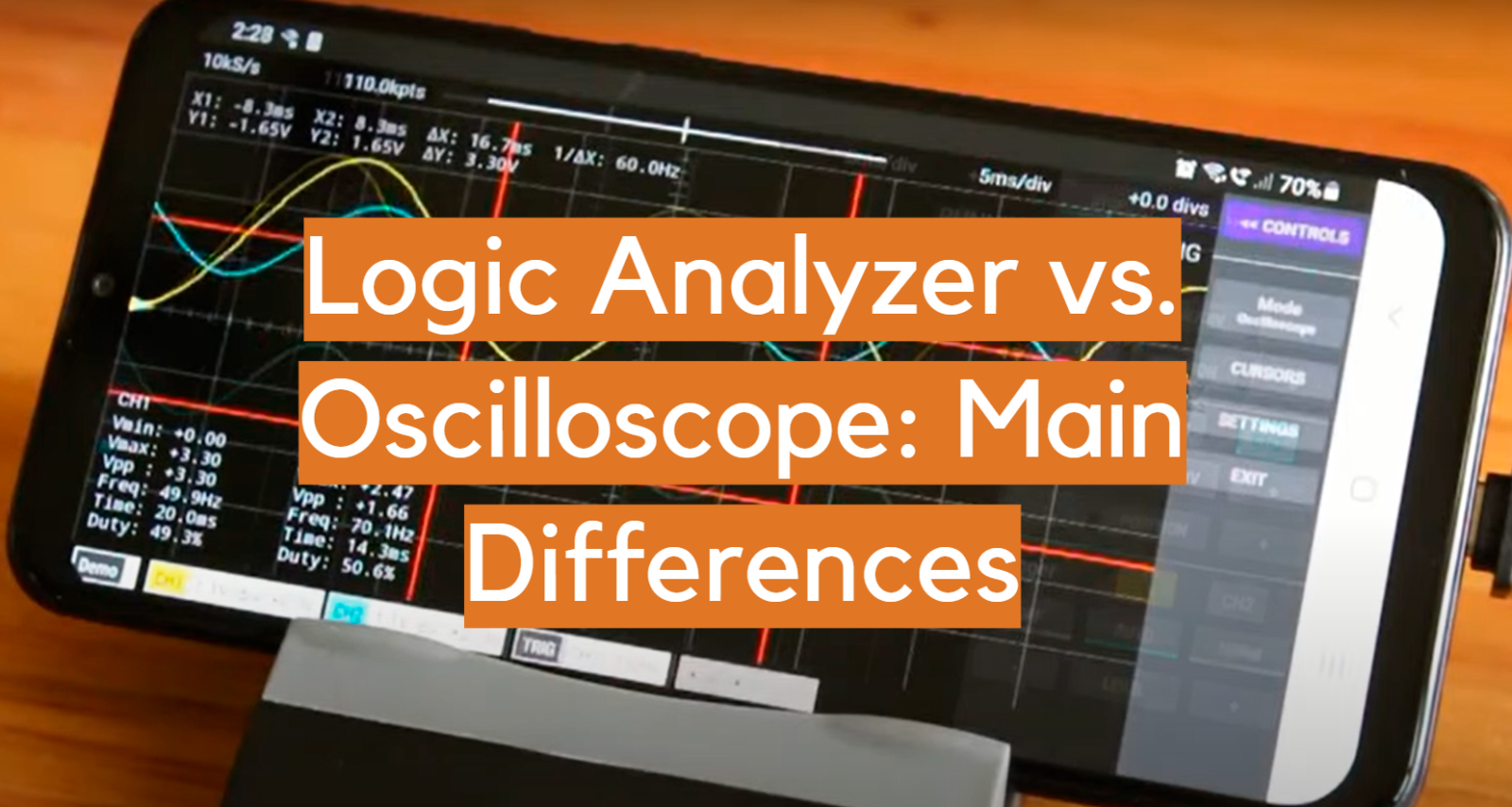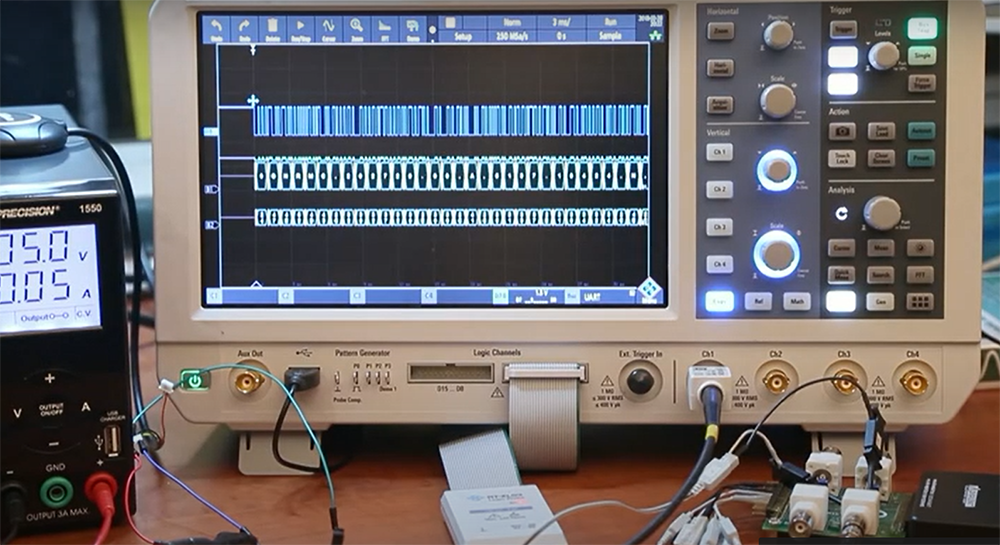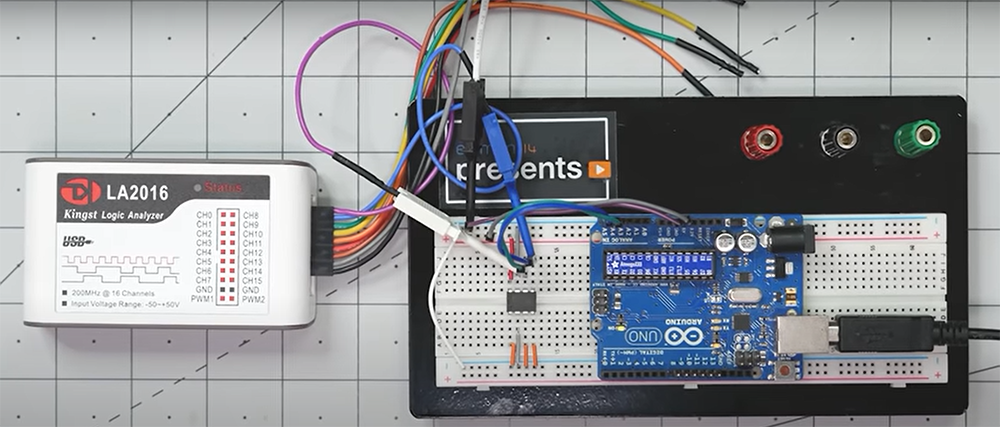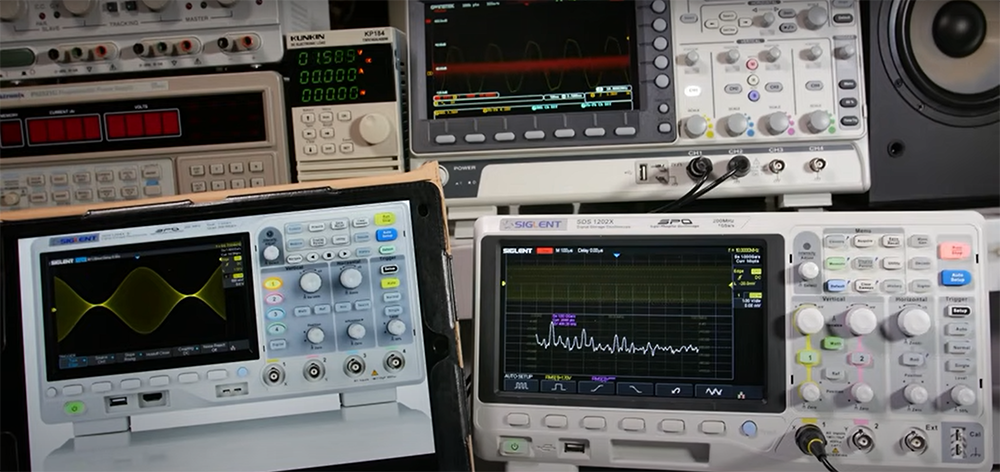# Logic Analyzer vs. Oscilloscope: Main DifferencesIf you are a hardware engineer, then you have probably heard of both the logic analyzer and the oscilloscope. But what are the main differences between these two devices? And which one is better for your needs? In this blog post, we will discuss the key differences between logic analyzers and oscilloscopes, and help you decide which device is best for your needs.

## What is a Logic Analyzer?

A logic analyzer is a device used to capture, decode and analyze digital signals. They are commonly used in digital design, embedded systems, and computer engineering.

It also helps in debugging digital circuits. The captured data can be browsed, searched, and analyzed offline or in real-time.

It works by sampling the input signal at regular intervals and storing the sampled values in its memory. Then, it displays the values in a waveform view .Logic analyzers come in two variants: digital and mixed-signal. The digital variant only captures and analyzes digital signals, while the mixed-signal variant can also capture and analyze analog signals.

They are available in different form factors, such as:

• Benchtop – These are the largest and most expensive type of logic analyzers. They are typically used in labs or workshops.
• Portable – These are smaller and lighter than benchtop logic analyzers, and can be easily carried around.
• USB – These are the smallest type of logic analyzers and can be plugged into a USB port on a computer.

## What is an Oscilloscope?

An oscilloscope is a device used to measure and display the waveforms of electrical signals. It usually has two vertical channels and one horizontal channel.

The two vertical channels are used to measure the amplitude of the signal, while the horizontal channel is used to measure the time period of the signal.

Oscilloscopes are typically used in electronic engineering to test and debug electronic circuits.

The working principle of an oscilloscope is based on the principle of time-domain reflectometry. It is able to measure voltage, current and time-domain parameters of an electrical signal.

They are available in different types, such as digital oscilloscopes and analog oscilloscopes.

• Digital oscilloscopes are able to sample the input signal and convert it into digital data. This data is then processed by a digital circuit and displayed on an LCD screen.
• Analog oscilloscopes use an electron beam to measure the voltage of an electrical signal. The amplitude of the signal is then converted into a brightness value, which is displayed on a CRT screen.

In addition, oscilloscopes can be either portable or benchtops. Portable oscilloscopes are small and lightweight, while benchtop oscilloscopes are larger and heavier.

## Difference between Logic Analyzer and Oscilloscope

There are many types of electronic test equipment but two of the most common are logic analyzers and oscilloscopes. While they may look similar, they have different purposes and use different techniques to measure signals.So let’s take a look at each one in turn and see what their main differences are.

### Uses

The first and most apparent difference between logic analyzers and oscilloscopes is their intended uses.

• Logic analyzers are used to capture, examine, and debug digital signals. Moreover, they are used to troubleshoot digital circuits and systems.
• Oscilloscopes, on the other hand, are used to measure and analyze electrical signals that vary with time. They are mainly used for circuit analysis and debugging electronics.

This means that logic analyzers are better suited for capturing data from digital devices such as microcontrollers, while oscilloscopes are better suited for troubleshooting problems with analog devices.

In addition, oscilloscopes can be used to measure frequency and phase, which logic analyzers cannot.

However, there is some overlap in their capabilities, and many logic analyzers also include oscilloscope functionality.

### Operation

The next main difference between a logic analyzer and an oscilloscope is how they operate.

• A logic analyzer samples data at a very high rate, usually many Megahertz, in order to capture the timing of digital signals. It also stores the data it samples in its memory.
• An oscilloscope operates by taking pictures of a waveform at discrete time intervals. This means that it can only show changes in voltage at those discrete time intervals. An oscilloscope samples data much more slowly, usually at a rate of only a few hundred kilohertz or less.

This slower sampling rate is not a problem for measuring analog signals, which is what an oscilloscope is designed to do. But it can be a problem for measuring digital signals, which can have very fast transitions.

The faster sampling rate of a logic analyzer is one of its main advantages over an oscilloscope. It can capture the timing of digital signals, which an oscilloscope cannot do. This can be important for troubleshooting digital circuits.

### Data Storage and Display

Logic analyzers typically have more data storage capacity than oscilloscopes. They also usually have a higher resolution, which means they can display more detail on the waveforms. However, oscilloscopes usually have a faster update rate, so they can show changes in the waveforms more quickly.Logic analyzers typically come with built-in software that makes it easy to view and analyze the data. Oscilloscopes usually require additional software to be installed on your computer in order to view the waveforms.

On the other hand, oscilloscopes typically have a more versatile range of inputs, which can be helpful for measuring signals that are not purely digital. Logic analyzers are better suited for analyzing digital signals only.

Logic analyzers often come with color displays, while oscilloscopes typically have black and white displays. This can be important if you need to see subtle differences in waveforms.

### Measurements

Logic analyzers and oscilloscopes are both used to measure voltage levels and waveforms, but there are some key differences between the two .

Oscilloscopes can only measure a single signal at a time, while logic analyzers can simultaneously measure multiple signals. Logic analyzers also offer more detailed data than oscilloscopes, making them better suited for measuring waveforms.

Logic analyzers are also better at detecting glitches and malfunctions, while oscilloscopes are better at displaying waveforms.

When choosing between a logic analyzer and an oscilloscope, consider how many signals you need to measure and what type of data you need.

If you need to measure multiple signals or detect glitches, a logic analyzer is the better option. If you need to see waveforms in detail, an oscilloscope is the better option.

### Trigger System

One more main difference between logic analyzers and oscilloscopes is trigger systems. Logic analyzers usually have a better trigger system than oscilloscopes.

• Most oscilloscopes have a trigger level that can be set by the user, which will cause the oscilloscope to start measuring data when the signal reaches that level. Oscilloscopes are designed to measure voltage levels, and as such, they can be easily triggered by changes in voltage levels.
• Logic analyzers usually have a pre-set trigger system that will cause them to measure data at specific points in time. This pre-set trigger system is usually more accurate than the trigger level on oscilloscopes. Logic analyzers are designed to capture digital signals, which means that they need a more sophisticated trigger system in order to capture data accurately.

The trigger system is important because it determines when data will be measured. If the wrong trigger setting is used, it can cause inaccurate data to be collected. The better the trigger system, the more accurate the data will be.

### Unique Features

You should also consider the unique features of each type of tool.

• Logic analyzers, for example, can trigger a specific event or state change, which can be helpful in debugging problems. It also has the ability to decode serial protocols, which can be very handy when trying to figure out what data is being transmitted between two devices.
• Oscilloscopes, on the other hand, typically have a faster update rate and can capture more signals at once. This makes them ideal for capturing transient waveforms, such as those found in electrical circuits. Oscilloscopes typically have more bandwidth than logic analyzers, making them better suited for high-speed signals.

In general, oscilloscopes are better at displaying time-domain signals, while logic analyzers are better at displaying state-based signals.

It’s important to consider what you’ll be using the tool for and which type of analyzer will best suit your needs. If you’re just starting out in electronics, a logic analyzer is probably the best place to start. But if you need to capture transient waveforms or debug serial protocols, an oscilloscope is the better option.

## Differences Between Logic Analyzers and Oscilloscopes: Comparison Table

While both of them serve similar purposes, they have significant differences in their functionality, features, and applications. This table presents a comparison of various indicators that differentiate logic analyzers and oscilloscopes.

Indicator Logic Analyzer Oscilloscope
Measurement Type Digital signals Analog signals
Sample Rate Higher Lower
Number of Channels Many Fewer
Data Analysis Deep, complex Shallow, quick
Price Higher Lower
Applications Design verification, protocol decoding Signal analysis, waveform visualization

The first indicator in the table is measurement type, which refers to the type of signals each device can measure. Logic analyzers are designed to measure digital signals, while oscilloscopes measure analog signals.

The second indicator is the sample rate, which is the number of measurements taken per second. Logic analyzers have a higher sample rate than oscilloscopes, which means they can capture more data in a shorter amount of time.

The number of channels is another important factor to consider. Logic analyzers typically have many channels, which allows them to capture a larger amount of data simultaneously. Oscilloscopes, on the other hand, have fewer channels.

The fourth indicator is triggering, which refers to the ability of the device to capture specific events or conditions. Logic analyzers have advanced triggering capabilities that allow them to capture complex events, while oscilloscopes have more basic triggering features.

Data analysis is another important factor to consider. Logic analyzers have the ability to perform deep, complex data analysis, while oscilloscopes have more shallow, quick analysis capabilities.

Price is another significant factor, with logic analyzers generally being more expensive than oscilloscopes.

Finally, the applications of each device differ. Logic analyzers are typically used for design verification and protocol decoding, while oscilloscopes are used for signal analysis and waveform visualization.

Check related articles to improve your knowledge in electronics:

## FAQ

### Can an oscilloscope measure resistance?

An oscilloscope can measure resistance if the circuit being tested is in series with a resistor of known value. However, it is not typically used for this purpose because a digital multimeter is better suited for this task.

### Is an oscilloscope more accurate than DMM?

No, an oscilloscope is not inherently more accurate than a digital multimeter. However, the accuracy of an oscilloscope can be improved by calibrating it using a known voltage or resistance. Additionally, many oscilloscopes include features that allow you to compensate for waveform distortion, which can improve their accuracy.

### Can an oscilloscope measure DC voltage?

Yes, an oscilloscope can measure DC voltage. However, its accuracy may be reduced when measuring very small voltages or when the waveform has a high frequency.

### Can an oscilloscope measure AC voltage?

An oscilloscope can measure AC voltage if it has an AC coupling feature. AC coupling removes the DC offset from the signal so that the oscilloscope can accurately measure the amplitude of the AC signal.

AC coupling is not available on all oscilloscopes, so check your product manual to see if your oscilloscope has this feature. If you need to measure AC voltage but don’t have an oscilloscope with AC coupling, you can use a rectifier to convert the AC signal into a DC signal.

### Can you use a logic analyzer as an oscilloscope?

Theoretically, yes – a logic analyzer can sample an analog signal just like an oscilloscope. However, in practice, this is rarely done because logic analyzers are more advanced tools designed for digital signals, while oscilloscopes are better equipped to handle analog signals.

Both tools have their pros and cons, so it really depends on what kind of problem you’re trying to solve.

### When would you use a logic analyzer over an oscilloscope?

The main difference between a logic analyzer and an oscilloscope is the type of signal that each one can measure. A logic analyzer can measure digital signals, while an oscilloscope is best used for measuring analog signals.

Oscilloscopes also allow you to view changes in real-time, while logic analyzers can take a snapshot of the signal at a specific point in time. If you need to see how a digital signal changes over time, then you would use a logic analyzer.

Logic analyzers allow you to see complex digital signals that may not be visible on an oscilloscope. They also tend to have more features and be more expensive than oscilloscopes.

### What is a mixed signal oscilloscope used for?

Mixed signal oscilloscopes (MSOs) are used in situations where it is necessary to observe and analyze both digital and analog signals at the same time. They offer many advantages over using a separate oscilloscope and logic analyzer, including the ability to see trigger events on both types of signals, measure frequency and amplitude relationships, and perform serial decoding.

Mixed signal oscilloscopes are used for a variety of applications where analog and digital signals need to be observed together. They are commonly used in embedded systems development, power analysis, automotive electronics design, and industrial control systems.

### What is a sampling oscilloscope?

A sampling oscilloscope is an electronic measuring instrument that converts an analog signal into a digital representation of that signal and then displays the waveform on a screen. Sampling oscilloscopes can be used to measure very fast signals, such as those generated by digital electronics. The main advantage of using a digital oscilloscope is that it can be connected to a computer, which allows for easy storage and analysis of the data.

### Can an oscilloscope measure frequency?

Yes, an oscilloscope can measure frequency. In fact, many oscilloscopes have a built-in frequency measurement function. To measure frequency with an oscilloscope, you simply need to configure the scope’s input voltage and timebase settings appropriately.

However, there are some limitations to using an oscilloscope for frequency measurement. First, the scope must be able to sample the signal at a high enough rate to accurately capture all of the signal’s cycles. Second, if the signal being measured is not periodic (i.e., it doesn’t repeat at regular intervals), then the scope will not be able to accurately measure its frequency.

For these reasons, it is often more convenient to use a dedicated frequency counter when measuring signals with an oscilloscope. A frequency counter is a specialized type of electronic test equipment that is designed specifically for measuring the frequency of electrical signals.

### What is the purpose of a logic analyzer?

The purpose of a logic analyzer is to provide a visual representation of digital signals so that you can troubleshoot and debug digital circuits. A logic analyzer is essentially a digital oscilloscope. It samples the input signal at regular intervals and displays the resulting waveform on a screen.

Logic analyzers are very useful for debugging complex digital circuits. They can be used to detect timing problems, glitches, and other anomalies that would be difficult to spot with a traditional oscilloscope.

### When would you consider using a logic analyzer for what kind of circuits?

Generally, a logic analyzer is used to monitor digital signals. This can be anything from simple TTL or CMOS levels, to more complicated protocols.

They are also useful if you have a board computer with a number of different digital buses going on, and you want to see what is happening on each one without having to physically probe every individual pin.

Another common use for them is in debugging microcontrollers. You can set breakpoints in the code, and then see exactly what state the various pins are in at that particular point in time. This can be invaluable when trying to track down elusive bugs.

In contrast, an oscilloscope is generally used for looking at analog signals. These could be voltages, currents, or even sound waves. They are less useful for looking at digital signals, although you can sometimes get away with it if the signal isn’t switching too fast.

### What are the advantages and disadvantages of a digital logic analyzer as compared to an oscilloscope?

There are several key advantages and disadvantages of digital logic analyzers as compared to oscilloscopes. First, let’s take a look at some of the advantages:

A digital logic analyzer usually has more channels to sample than an oscilloscope – this means that you can monitor more digital signals at the same time. They also often have deeper memory buffers, meaning that you can capture longer events and save them for later analysis.

Logic analyzers also allow you to measure time event capture points – so you can see not just when an event occurred, but also how long it took for another event to happen afterwards. This can be very useful for troubleshooting digital circuits.

Now let’s look at some of the disadvantages:

One downside of logic analyzers is that they don’t display signals in real-time – you have to trigger the start of the sampling, and then wait for it to finish before you can see the results. Oscilloscopes, on the other hand, display signals in real-time.

Another downside is that logic analyzers can’t measure analog characteristics of signals like oscilloscopes can. This means that if you want to measure things like voltage or current, you’ll need to use an oscilloscope.

### What are the limitations of an oscilloscope?

An oscilloscope is a very powerful tool, but it has its limitations. One of the biggest limitations is that it can only measure one signal at a time. This means that if you want to measure multiple signals, you have to use multiple oscilloscopes or take turns measuring each signal.

Another limitation of an oscilloscope is that it can only measure analog signals. This means that if you want to measure digital signals, you have to use a logic analyzer.

Finally, oscilloscopes can be expensive. If you only need to measure one signal, an oscilloscope is probably overkill and you would be better off with a less expensive tool like a multimeter.

### What is the minimum frequency oscilloscope?

The minimum frequency an oscilloscope can measure is determined by its bandwidth. Bandwidth is the difference between the highest and lowest frequencies an instrument can accurately reproduce. Most general-purpose oscilloscopes have a bandwidth of at least 50 Hz, which lets you see signals with frequencies up to 50 Hz without distortion.

Oscilloscopes draw 1 Hz per one second so 50 Hz bandwidth means that the oscilloscope can draw 50 times per second.

### What is the accuracy of an oscilloscope?

While accuracy isn’t that important of a factor when comparing a logic analyzer vs oscilloscope, it is still worth mentioning. The accuracy of an oscilloscope is around 3% to 5% off from the real value. This is due to the fact that an oscilloscope measures voltages and not time, so there is a slight margin of error.

For more accurate measurements, you will need to use a multimeter. However, for most purposes, an oscilloscope is accurate enough.

## Final Thoughts

Logic analyzers and oscilloscopes are both common tools in electronic engineering, but they have some key differences.

The most obvious is that a logic analyzer captures digital signals, while an oscilloscope captures analog signals. Additionally, a logic analyzer typically has more channels than an oscilloscope.

However, the most important difference is that a logic analyzer can decode digital signals, while an oscilloscope cannot. This means that a logic analyzer can tell you what each bit in a signal means, while an oscilloscope just shows you the waveform.

This makes the logic analyzer much more useful for debugging digital circuits, while the oscilloscope is better for debugging analog circuits.

I hope this article has helped you understand the main differences between logic analyzers and oscilloscopes. Thanks for reading!